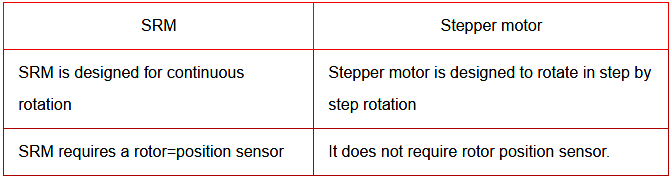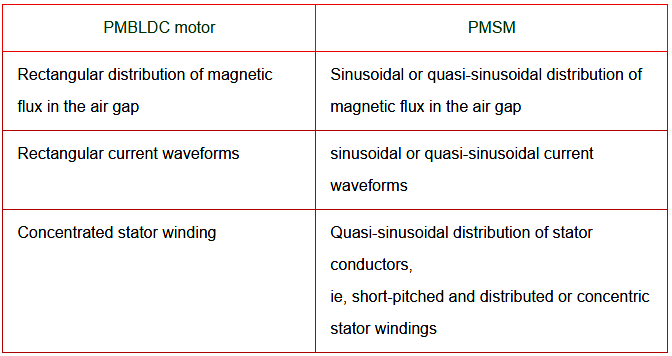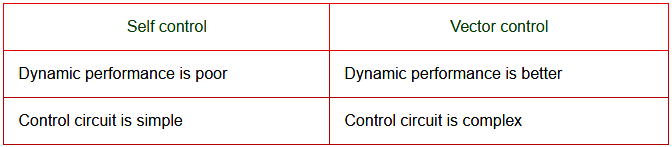Home » Electrical Drives and Controls Interview Questions
Electrical Q & A

Electrical Drives and Controls Interview Questions

 What is Switched Reluctance Motor?

The switched reluctance motor is a double salient, singly excited motor. This means that it has salient pole on the rotor and the stator, but only one member carries windings. The rotor has no windings, magnets or cage windings. It works on variable reluctance principle.

 What are the two types of control techniques in SRM?

1. Hysteresis type control
2. PWM type control

 What is meant by energy ratio?

Energy ratio = Wm/[Wm + R] = 0.455
Wm = mechanical energy transformed
This energy ratio cannot be called as efficiency. As the stored energy R is not wasted as a loss but it is fed back to the source through feedback diodes.

 What is phase wingdings?

Stator poles carrying field coils. The field coils of opposite poles are connected in series such that mmfs are additive and they are called phase wingdings of SRM

 What are the major difference between SRM and stepper motor?What is hysteresis current control?

This type of current controller maintains a more or less constant current throughout the conduction period in each phase. This controller is called hysteresis type controller.

 When do you go for chopping mode of control in switched reluctance motor?

The chopping mode of control in SRM is applied only low-speed. Here each phase winding gets excited for a period which is sufficiently long.

 What is the working principle of Switching Reluctance Motor?

The switched reluctance motor basic principle is based on Faraday’s law of electro magnetic interaction.

• Whenever a current carrying conductor is placed in a magnetic field, it experiences a force. The direction of force given by Fleming’s left hand rule.
• SRM is a single excited and double salient pole electric motor. Due to the variation of reluctance the motor should be operated.
• The SRM develops a torque due to variable reluctance.
• When air gap is minimum, the reluctance will be minimum; hence inductance will be maximum.
• So the rate of change of inductance is zero.
• When the reluctance various in the motor, there will be a change in inductance so when a particular stator winding of SRM is energized,the rotor pole comes in alignment with that stator pole and thus the rotor rotates.

 Why rotor position sensor is essential for the operation of Switched Reluctance Motor?

It is normally necessary to use a rotor position sensor for commutation and speed feed back. The turning ON and OFF operation of the various devices of power semiconductor switching circuit are influenced by signals obtained from rotor position sensor.

 List the disadvantages of a Switched Reluctance Motor?

• Stator phase winding should be capable of carrying magnetizing current.
• For high-speed operation developed torque has undesirable ripples is a result develops undesirable noises or acoustic noises.
• For high-speed current waveform has undesirable harmonics to suppress this effect large size capacitor is to be connected.
• It requires position sensors.

 What are the advantages of switched reluctance motor?

1. Construction is simple and robust.
2. Rotor carries no windings, no slip rings, no brushes, less maintenance.
3. There is no permanent magnets.
4. Ventilating system is simpler as losses takes place mostly in the stator.
5. Power semiconductor switching circuitry is simpler.
6. No shoot through fault likely to happen power short circuits.
7. Developed torque does not depends upon the polarity of current in the phase winding.
8. The operation of the machine can be easily change from motoring mode to generating mode by varying the region of conduction.
9. It is possible to get very high-speed.
10. Depending upon the requirement T-w characteristics can be achieved.
11. It is the self-starting machine.
12. Energy stored in the phase winding is fed back to the supply through the feedback diodes during off period.

 Whar are the applications of SRM?

1. Washing machines
2. Vacuum cleaners
3. Fans
4. Future auto mobile applications
5. Robotics control applications

 What is the difference between Synchronous Reluctance Motor and PM Synchronous Motor?What are the differences between PMBLDC and PMSM?Distinguish between self-control and vector control of PMSMWhat is meant by slotless motor?

In slotless motor, the stator teeth are removed and resulting space is partially filled with addition of copper

 State the advantages of PMSM?

1. Improved performance
2. Reliability increases
3. Reduced components
4. Versatility of the controller
5. Less cost

1. It runs at constant speed.
2. No field winding, no field loss, better efficiency.
3. No sliding contacts, so it requires less maintenance.

1. Power factor of operation can not be controlled as field winding cannot be controlled.
2. It leads to losses and decreases efficiency

 Write the EMF equation of PMSM

Eph = 4.44 f. Φm. Kp. Kb. Nph volts

This is the RMS value of induced EMF per phase
where
f – frequency in Hz
Nph – Turns per phase
Φm – Flux per pole
Kw – Winding factor

 What are the assumptions made in derivation of EMF equation for PMSM?

1. Flux density distribution in the air gap is sinusoidal.
2. Rotor rotates with a uniform angular velocity of ωm(r/sec).
3. Armature winding consists of full pitched, concentrated similarly located coils of equal number of turns.

 Why PMSM operating in self-controlled mode is known as Commutatorless DC Motor?

Load side controller performs somewhat similar function as commutator in a DC machine. The load side converter and synchronous motor combination functions similar to a DC machine.

First it is fed from a DC supply and secondly like a DC machine. The stator and rotor field remain stationary with respect to each other at all speeds. Consequently, the drive consisting of load side converter and synchronous motor is known as commutator less DC motor.

 What is Pulse Mode?

• For speeds below 10% of base speed, the commutation of load side converter SCRs is done by forcing the current through the conducting SCRs to zero.
• This is realised by making source side converter to work as inverter each time load side converter SCRs are to be turned off.
• Since the frequency of operation of load side converter is very low compared to the source frequency, such kind of operation can be realised.
• The operation of inverter is termed as Pulsed Mode.

Commutation of thyristors by induced voltages of load is known as Load Commutation. Here, frequency of operation is higher and it does not require commutation circuits.

 What is meant by self-control?

• As the rotor speed changes the armature supply frequency is also changes proportionally so that the armature field always moves (rotates) at the same speed as the motor.
• The armature and rotor field move in synchronism for all operating points.
• Here accurate tracking of speed by frequency is realised with the help of rotor position sensor.